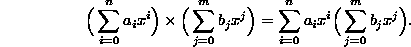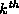Data Structures and Algorithms with Object-Oriented Design Patterns in C## Projects

1. Write a visitor to solve each of the following problems:
1. Find the smallest element of a list.
2. Find the largest element of a list.
3. Compute the sum of all the elements of a list.
4. Compute the product of all the elements of a list.
2. Design and implement a class called OrderedListAsDoublyLinkedList which represents an ordered list using a doubly-linked list. Select one of the approaches shown in Figure.
3. Consider the Polynomial class given in Program. Implement a method that computes the value of a polynomial, say p(x), for a given value of x. Hint: Use a visitor that visits all the terms in the polynomial and accumulates the result.
4.   Devise and implement an algorithm to multiply two polynomials. Hint: Consider the identityWrite a method to multiply a Polynomial by a Term and use the polynomial addition operator defined in Program.

5. Devise and implement an algorithm to compute thepower of a polynomial, where k is a positive integer. What is the running time of your algorithm?
6. For some calculations it is necessary to have very large integers, i.e., integers with an arbitrarily large number of digits. We can represent such integers using lists. Design and implement a class for representing arbitrarily large integers. Your implementation should include operations to add, subtract, and multiply such integers, and to compute thepower of such an integer, where k is a small positive integer. Hint: Base your design on the Polynomial class given in Program.Copyright © 2001 by Bruno R. Preiss, P.Eng. All rights reserved.